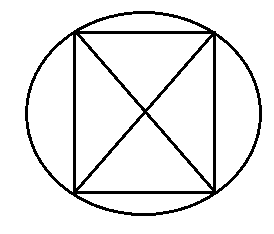#### All the vertices of a rhombus lie on a circle. Find the area of the rhombus, if area of the circle is 1256 cm2. (Use = 3.14).

SolutionGiven that area of circle =1256cm2

Diameter of circle = 40 cm

As we know that the diameter of circle is equal

Diagonals of rhombus = Diameters of circle = 40 cm

Each diagonals of rhombus = 40 cm

Area of rhombus =  product of digonals

=   40 40

= 800cm2

Hence required area of rhombus is =800cm2